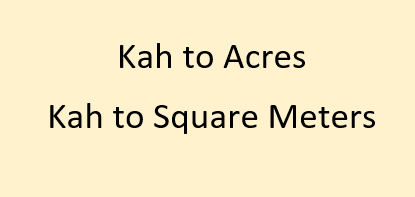# Kah to Acres | Kah to Square Meters## Kah to Acres | Kah to Square MetersKah is a traditional unit of land area measure which is still commonly used in Taiwan. It is derived from Dutch Morgen when Taiwan was under Dutch era. it is also known as Kap in Hakka and Jia in Mandarin.Its Character is 甲. Being a traditional unit of people wish to know the equivalent value in standard units of measurement so they ask questions like 1 Kah is how many acres or kah is equal to how many square Meters ?
1 Kah is equal to 2934 Pe
so, 1 Kah = 1173600/121 square meters
1 Kah = 9699.17355 Square Meters (approx) = 2.3967 Acres (approx)

Convert Kah to other units of Land area measurement :
 Value Convert from Le Kah Hun Bo Pe Sq. Centimeters Sq. Feet Sq. Yards Hectares Acres Sq. Meters Sq. Kilometers Sq. Miles Cent Convert to Le Kah Hun Bo Pe Sq. Centimeters Sq. Feet Sq. Yards Hectares Acres Sq. Meters Sq. Kilometers Sq. Miles Cent

Area Conversion of Kah to other Units and vice versa:
1 Kah = 2.39671798090798 Acre
1 Kah = 0.969917355371901 Hectare
1 Kah = 104401.035248351 Sq. Feet
1 Kah = 9699.17355371901 Sq. Meter
1 Kah = 0.00969917355371901 Sq. Kilometer
1 Kah = 11600.1150275946 Sq. Yard
1 Kah = 239.671798090798 Decimal
1 Kah = 0.2 Le
1 Kah = 10 Hun
1 Kah = 97.8 Bo
1 Kah = 2934 Pe
1 Le = 5 Kah
1 Hun = 0.1 Kah
1 Bo = 0.0102249488752556 Kah
1 Pe = 0.000340831629175187 Kah
1 Acre = 0.41723724191411 Kah
1 Hectare = 1.03101567825494 Kah
1 Sq. Feet = 9.5784490797546E-06 Kah
1 Sq. Meter = 0.000103101567825494 Kah
1 Sq. Kilometer = 103.101567825494 Kah
1 Sq. Yard = 8.62060417177914E-05 Kah
1 Decimal = 0.0041723724191411 Kah

Q. 1 Acre consists of how many Kah
A. 1 Acre consists of approx 0.417 Kah

Q. Convert Kah to Square Yards
A. 1 Kah is equal to approx 11600 Square Yards

Q. Kah to other traditional units of land measurement
A. 1 Kah = 0.2 Le = 10 Hun = 97.8 Bo = 2934 Pe

Q. 1 Kah is how many sq feet?
A. 1 Kah = 104401.035248351 Sq. Feet

Q. 1 Hectare is how many Kah?
A. 1 Hectare = 1.03101 Kah(approx)